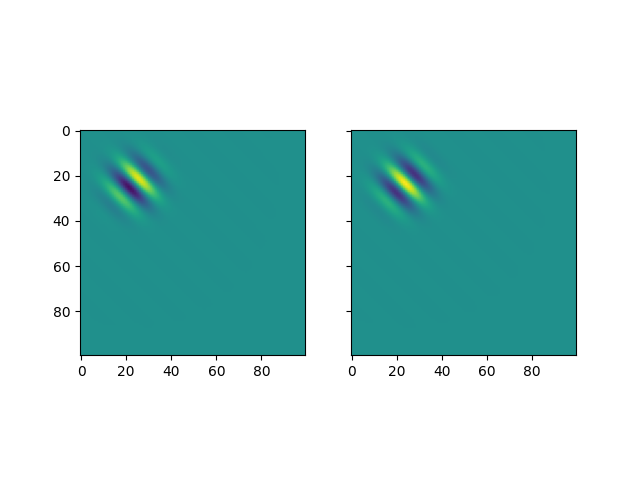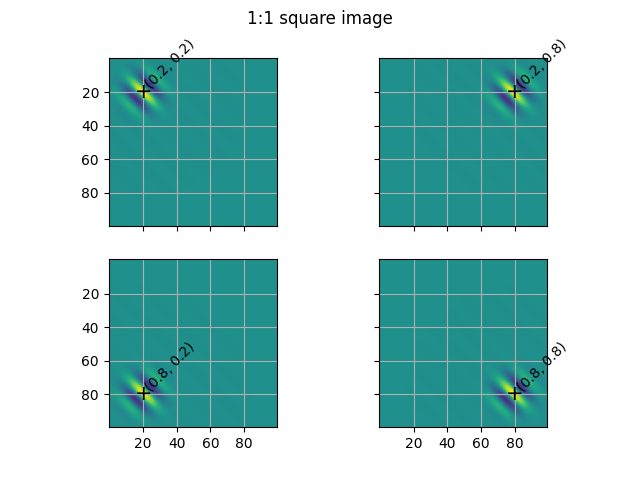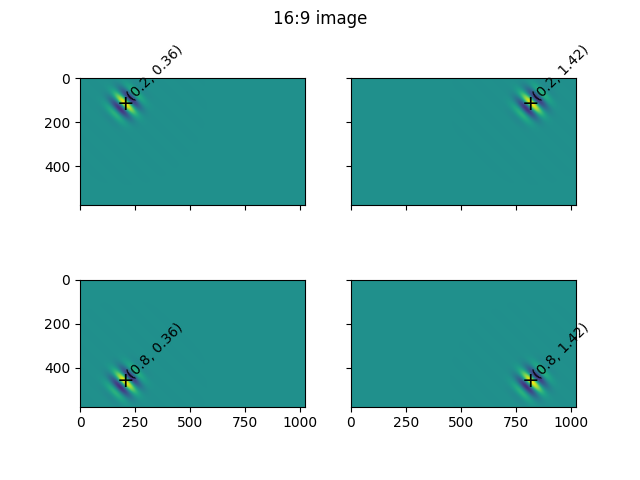# Image coordinates¶

Description of the image coordinates used to define the motion energy filters.

Coordinates are specified as (vertical, horizontal).

• (0,0): top-left

• (1,0): bottom-left

• (0,aspect_ratio): top-right

• (1,aspect_ratio): bottom-right

## 1:1 square aspect ratio¶

import moten
pyramid = moten.get_default_pyramid(vhsize=(100,100), fps=24)


The vertical and horizontal positions of each filter are stored as centerv and centerh, respectively, in the filter dictionary:

from pprint import pprint
example_filter = pyramid.filters.copy()
pprint((example_filter['centerv'], example_filter['centerh']))


Out:

(0.2375, 0.2375)


This is what the spatial components of this filter look like. (Note that we will only visualize the cosine component in the rest of this example).

import numpy as np
import matplotlib.pyplot as plt
fig, axes = plt.subplots(ncols=2, sharex=True, sharey=True)
_ = axes.imshow(ssin, vmin=-1, vmax=1)
_ = axes.imshow(scos, vmin=-1, vmax=1)We can move this filter to different positions in the image. Each filter has the parameters centerh and centerv. When both are zero, the filter is located at the top left corner of the image. To move the filter to different positions, all we need to do is update the centerh and centerv parameters in the filter dictionary.

The position parameters are defined in terms of percentages of height. Below, we define 4 new positions located at a 20% distance from the top, bottom, left and right.

vh_positions = [(0.2, 0.2), # top left
(0.2, 0.8), # top right
(0.8, 0.2), # bottom left
(0.8, 0.8), # bottom right
]

fig, axes = plt.subplots(nrows=2, ncols=2, sharex=True, sharey=True)
for idx, (vpos, hpos) in enumerate(vh_positions):
axidx = np.unravel_index(idx, axes.shape)
ax = axes[axidx]

# Here we update the position of the filter
modified_filter = example_filter.copy()
modified_filter['centerv'] = vpos
modified_filter['centerh'] = hpos

# Show the filter
ax.imshow(scos, vmin=-1, vmax=1.0)

# Label its position
vsize = pyramid.definition['vdim']
ax.text(hpos*vsize, vpos*vsize, '+', va='center', ha='center', fontsize=15)
ax.text(hpos*vsize, vpos*vsize, (vpos, round(hpos,2)),
va='bottom', ha='left', rotation=45)
ax.set_xticks([20, 40, 60, 80])
ax.set_yticks([20, 40, 60, 80])
ax.grid(True)

_ = fig.suptitle('1:1 square image')## 16:9 aspect ratio¶

In the example above, the width and height of the image is the same (100x100 pixels). For most videos, this is not the case. Videos typically have a width/height aspect ratio of 4:3 (e.g. 800x600) or 16:9 (e.g. 1920x1080). Below, we define a pyramid with a 16:9 aspect ratio.

pyramid = moten.get_default_pyramid(vhsize=(576 , 1024), fps=24)
vsize = pyramid.definition['vdim']


We update the aspect ratio of the example filter to match the new pyramid.

aspect_ratio = pyramid.definition['aspect_ratio']
assert np.allclose(aspect_ratio, 16/9)
example_filter['aspect_ratio'] = aspect_ratio


This 16:9 aspect ratio means that the right-most point of the image is (16/9)*height. In order to position filters at the desired 20% and 80% horizontal positions, we need to scale the horizontal dimension by the aspect ratio:

vh_positions = [(0.2, aspect_ratio*0.2), # top left
(0.2, aspect_ratio*0.8), # top right
(0.8, aspect_ratio*0.2), # bottom left
(0.8, aspect_ratio*0.8), # bottom right
]


Now, we can plot the filters at the desired positions using the same code:

fig, axes = plt.subplots(nrows=2, ncols=2, sharex=True, sharey=True)
for idx, (vpos, hpos) in enumerate(vh_positions):
axidx = np.unravel_index(idx, axes.shape)
ax = axes[axidx]

modified_filter = example_filter.copy()
modified_filter['centerv'] = vpos
modified_filter['centerh'] = hpos

ax.imshow(scos, vmin=-1, vmax=1.0)

vsize = pyramid.definition['vdim']
ax.text(hpos*vsize, vpos*vsize, '+', va='center', ha='center', fontsize=15)
ax.text(hpos*vsize, vpos*vsize, (vpos, round(hpos,2)), va='bottom', ha='left', rotation=45)

_ = fig.suptitle('16:9 image')

# sphinx_gallery_thumbnail_number = 2Total running time of the script: ( 0 minutes 0.843 seconds)

Gallery generated by Sphinx-Gallery